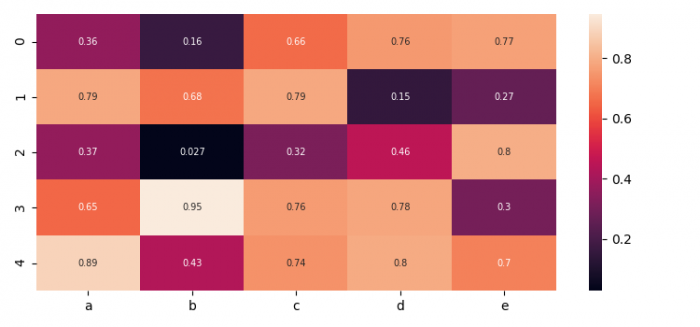# How to annotate each cell of a heatmap in Seaborn?

To annotate each cell of a heatmap, we can make annot = True in heatmap() method.

## Steps

• Set the figure size and adjust the padding between and around the subplots.
• Create a Pandas dataframe with 5 columns.
• Use sns.heatmap() to plot a dataframe (Step 2) with annot=True flag in the argument.
• To display the figure, use show() method.

## Example

import seaborn as sns
import pandas as pd
import numpy as np
import matplotlib.pyplot as plt
plt.rcParams["figure.figsize"] = [7.50, 3.50]
plt.rcParams["figure.autolayout"] = True
df = pd.DataFrame(np.random.random((5, 5)), columns=["a", "b", "c", "d", "e"])
sns.heatmap(df, annot=True, annot_kws={"size": 7})
plt.show()

## Output